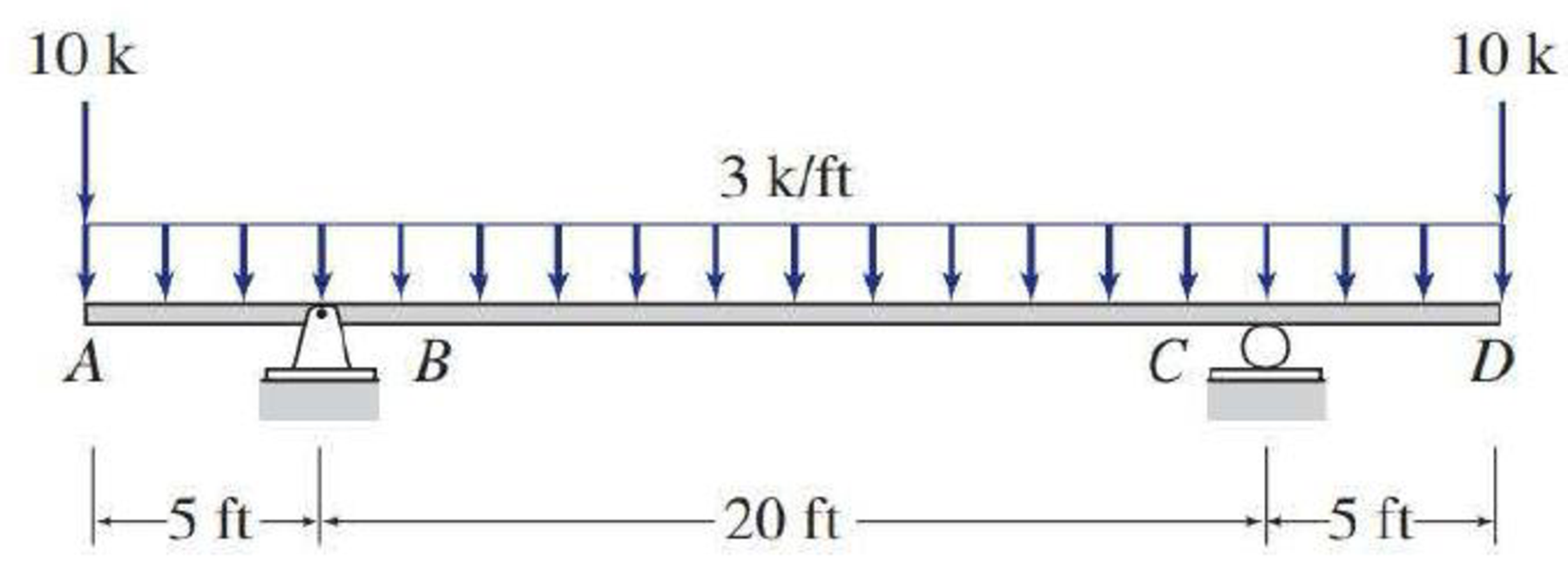# 5.29 through 5.51 Draw the shear and bending moment diagrams and the qualitative deflected shape for the beam shown.

#### Solutions

Chapter
Section
Chapter 5, Problem 41P
Textbook Problem
250 views

## 5.29 through 5.51 Draw the shear and bending moment diagrams and the qualitative deflected shape for the beam shown.To determine

Plot the shear and bending moment diagram and the qualitative deflected shape.

### Explanation of Solution

Sign conversion:

Apply the sign convention for calculating the equations of equilibrium as below.

• For the horizontal forces equilibrium condition, take the force acting towards right side as positive (+) and the force acting towards left side as negative ().
• For the vertical forces equilibrium condition, take the upward force as positive (+) and downward force as negative ().
• For moment equilibrium condition, take the clockwise moment as negative and counter clockwise moment as positive.

Apply the following sign convention for calculating the shear and bending moments.

• When the portion of the beam considered is left of the section, then the external force acting to the left are considered as positive.
• When the portion of the beam considered is right of the section, then the external force acting to the right are considered as positive.
• When the portion of the beam considered is left of the section, then the external force acting upward are considered as positive.
• When the portion of the beam considered is right of the section, then the external force acting downward are considered as positive.
• When the portion of the beam considered is left of the section, then the clockwise moments are considered as positive.
• When the portion of the beam considered is right of the section, then the counterclockwise moments are considered as positive.

Calculation:

Show the free-body diagram of the entire beam as in Figure 1.

Find the horizontal reaction at point B by resolving the horizontal equilibrium.

+Fx=0Bx=0

Find the vertical reaction at point C by taking moment about point B.

+MB=010(5)+3(5)(52)3(25)(252)10(25)+Cy(20)=050+37.5937.5250+20Cy=0Cy=55k

Find the vertical reaction at point B by resolving the vertical equilibrium.

+Fy=010+By3(30)10+Cy=010+By9010+55=0By=55k

Show the reactions of the beam as in Figure 2.

### Still sussing out bartleby?

Check out a sample textbook solution.

See a sample solution

#### The Solution to Your Study Problems

Bartleby provides explanations to thousands of textbook problems written by our experts, many with advanced degrees!

Get Started

Find more solutions based on key concepts
List five products that are not currently on the market, which could be useful to us and will most likely be de...

Engineering Fundamentals: An Introduction to Engineering (MindTap Course List)

Have you been following the above guidelines while using mobile computers and devices? If not, what have you be...

Enhanced Discovering Computers 2017 (Shelly Cashman Series) (MindTap Course List)

What is total cost of ownership (TCO)? What costs often are underestimated?

Systems Analysis and Design (Shelly Cashman Series) (MindTap Course List)

Explain the difference between a machining center and a CNC mill.

Precision Machining Technology (MindTap Course List)

If your motherboard supports ECC DDR3 memory, can you substitute non-ECC DDR3 memory?

A+ Guide to Hardware (Standalone Book) (MindTap Course List)